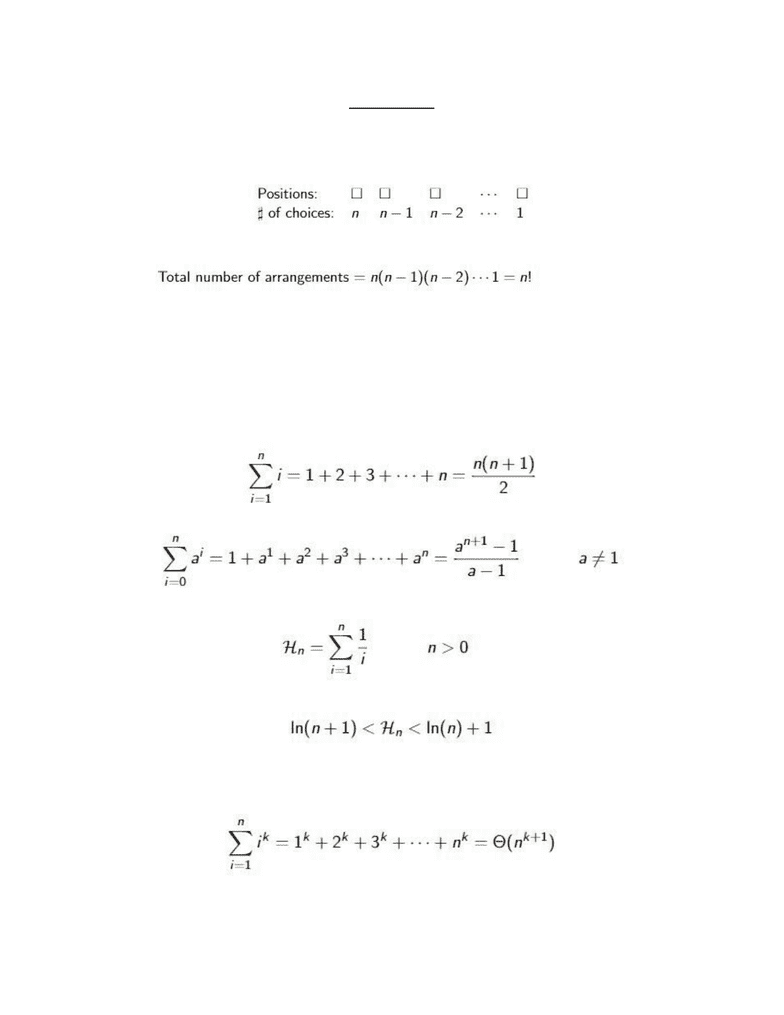# COMPENG 2SI4 Lecture 2: Math Review and Binary Search

35 views3 pages
School
McMaster University
Department
Computer Engineering
Course
COMPENG 2SI4Math Review
Permutations
Ordered arrangement of a number of objects
If there are n distinct elements there are n! Permutations
Modular Arithmetic
The modulus function returns the remainder after dividing two integer
Say we have 10 modulo 8 → this gives an answer of 2
In java this operation is written as 10%8
Arithmetic and Geometric Series
These series are good to know
Harmonic Series
The above series diverges as n goes to infinity but it can be shown that:
And therefore we can say that the series diverges logarithmically as n approaches infinity
Sum of Consecutive Numbers Raised to the Same Power
Unlock document

This preview shows page 1 of the document.
Unlock all 3 pages and 3 million more documents.